# Formulas

COLLAPSE PRESSURE

For Minimum Collapse Failure in the Plastic Range with Minimum Yield Stress Limitations: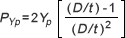Applicable D/t Span

 API GRADE D/T RATIO SPAN H-40 casing and tubing 16.40 and less K-55 casing and J-55 tubing 14.81 and less C-75 casing and tubing 13.60 and less L-80 and N-80 casing and tubing 13.38 and less C-90 casing and tubing 13.01 and less C-95 casing, X-95 drill pipe, and T-95 casing and tubing 12.85 and less P-105 tubing 12.57 and less P-110 casing 12.44 and less Q-125 casing 12.11 and less

For Minimum Failure in the Plastic Range: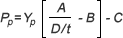Factors and Applicable D/t Span

 API GRADE A B C D/T RATIO SPAN H-40 2.950 .0465 754 16.40 to 27.01 J-55 and K-55 2.991 .0541 1206 14.81 to 25.01 C-75 and E 3.054 .0642 1806 13.60 to 22.91 L-80 and N-80 3.071 .0667 1955 13.38 to 22.47 C-90 3.106 .0718 2254 13.01 to 21.69 C-95 3.124 .0743 2404 12.85 to 21.33 P-105 3.162 .0794 2702 12.57 to 20.70 P-110 3.181 .0819 2852 12.44 to 20.41 Q-125 3.239 .0895 3301 12.11 to 19.63

For Minimum Failure in Transition Zone Between Plastic and Elastic Range: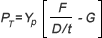Factors and Applicable D/t Ratio Span

 API GRADE F G D/T RATIO SPAN H-40 2.063 .0325 27.01 to 42.64 J-55 and K-55 1.989 .0360 25.01 to 37.21 C-75 1.990 .0418 22.91 to 32.05 L-80 and N-80 1.998 .0434 22.47 to 31.02 C-90 2.017 .0466 21.69 to 29.18 C-95 2.029 .0482 21.33 to 28.36 P-105 2.053 .0515 20.70 to 26.89 P-110 2.066 .0532 20.41 to 26.22 Q-125 2.106 .0582 19.63 to 24.46

For Minimum Failure in the Elastic Range:Applicable D/t Span

 GRADE D/T RATIO SPAN H-40 42.64 and greater J-55 and K-55 37.21 and greater C-75 32.05 and greater L-80 and N-80 31.02 and greater C-90 29.18 and greater C-95 28.36 and greater P-105 26.89 and greater P-110 26.22 and greater Q-125 24.46 and greater

Yp = Minimum Yield Strength

 GRADE FACTORS IN PSI H-40 40,000 J-55 and K-55 55,000 C-75 75,000 L-80 and N-80 80,000 C-90 90,000 C-95 95,000 P-105 105,000 P-110 110,000 Q-125 125,000 S-135 135,000

Where: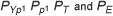= Minimum collapse pressure in psi D = Nominal outside diameter in inches t = Nominal wall thickness in inches

Collapse Pressure Under Axial Tension Stress: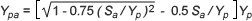Minimum collapse pressure under axial load can be determined from tables in API Bul 5C2, 20th Edition, 1987.

INTERNAL PRESSURE

Internal Yield Pressure
(Casing, Tubing and Drill Pipe – Minimum Wall)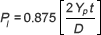Where:

 Pl = minimum internal yield pressure in psi D = nominal outside diameter of pipe in inches t = nominal wall thickness of pipe in inches Yp = specified minimum yield strength in psi

Internal Pressure of pipe at 80% of Minimum Yield:
(Also: Maximum Formation Fracturing Pressure)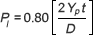Internal Pressure of Couplings: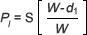Where:

 S = 80% of Yp for mill test pressure and for maximum fracturing pressure = 100% of Yp for internal yield pressure W (W1 for special clearance) = outside diameter of coupling d1 = thread root diameter of coupling at end of pipe in power-tight position

For round thread casing and tubing couplings:Where:
T = included taper on diameter

Other symbols are listed in API Std. 5B.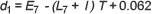Where:

 T = included taper on diameter l = 0.400 in. for 41/2 in. OD = 0.500 in. for 5 in. through 133/8 in. OD = 0.375 in. for 16 in. OD and larger

Other symbols are listed in API Std.5B.

Internal Pressure Leak Resistance: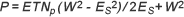Where:

 P = internal pressure, psi E = 30 x 106 T = thread taper N = no. of thread turns make-up p = thread pitch W = coupling OD Es = E1 for round thread = E7 for buttress thread

JOINT STRENGTH

The following equations apply to both short and long threads and couplings. One is for failure by fracture and the other is for failure by thread jumping or pullout; the lesser of the resulting values is listed as the joint strength.

Fracture Strength: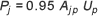Pullout Strength: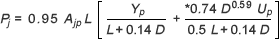Where:

 Pj = minimum joint strength in pounds Ajp = cross-sectional area of the pipe wall under the last perfect thread, in square inches = 0.7854 [ ( D – 0.1425)2 –d2 ] D = nominal outside diameter of pipe in inches d = nominal inside diameter of pipe in inches L = engaged thread length in inches = L4 – M for nominal make-up, as listed in API Std. 5B Yp = minimum yield strength of pipe in pounds per square inch Up = minimum ultimate strength of pipe in pounds per square inch

 D *.74 D 0.59 4 1/2 .30467 5 .28631 5 1/2 .27066 6 5/8 .24251 7 .23476 7 5/8 .22321 8 5/8 .20756 9 5/8 .19455 10 3/4 .18226 11 3/4 .17295 13 3/8 .16022 16 .14415 18 5/8 .13179 20 .12636

For casing with buttress threads, two formulas are used; one is for the strength of the threaded pipe and the other, for the coupling. The lesser of the values obtained by the two formulas governs.

Pipe Strength: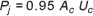Where:

 Pj = minimum joint strength in pounds Yp = minimum yield strength of pipe, psi Up = minimum ultimate strength of pipe, psi Uc = minimum ultimate strength of coupling, psi Ap = cross sectional area of pipe, square inches .7854 ( D2 – d2 ) Ac = cross sectional area of coupling, square inches .7854 ( W2 – d12 ) D = outside diameter of pipe, inches d = inside diameter of pipe, inches W = outside diameter of coupling, inches d1 = diameter at the root of the coupling thread at the end of the pipe in the power-tight position

Drill Pipe and Round Thread Tubing

Yield strength values for round thread tubing and drill pipe are determined as the product of the specified minimum yield strength for the steel grade and the area of section under the root of the last perfect thread of the pipe or of the area of the body of the pipe, whichever is smaller.

Non-Upset Tubing: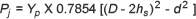External Upset Tubing and Internal and External Upset Drill Pipe:Where:

 Pj = minimum joint strength in pounds D = nominal outside diameter of pipe in inches d = nominal inside diameter of pipe in inches hs = = = height of thread in inches 0.05560 in. for 10 threads per inch 0.07125 in. for 8 threads per inch Yp = specified minimum yield strength of pipe in pounds per square inch. Values are listed elsewhere.

Folrmulas